Radio Shack TRS-80 Pocket Computer PC-4

Line of Position Program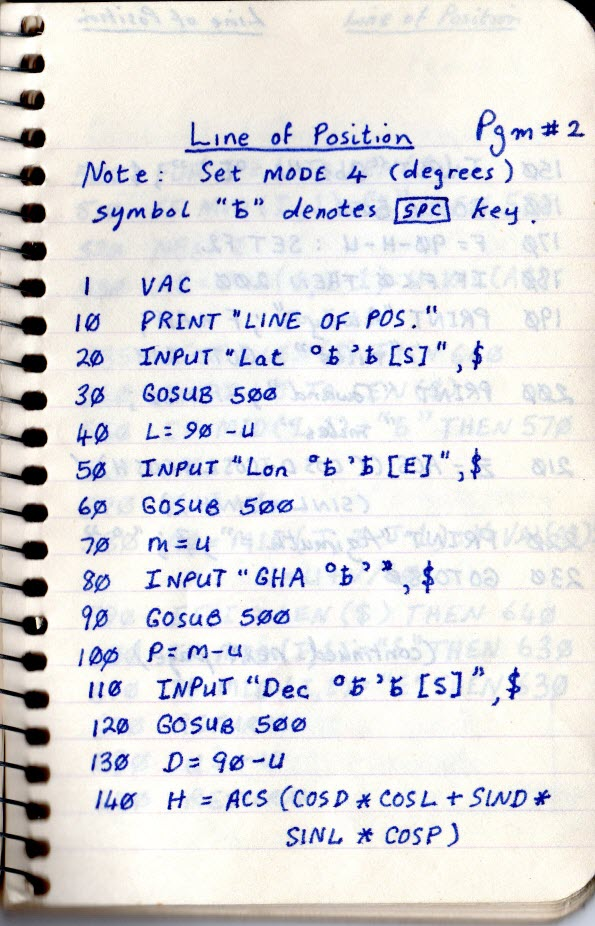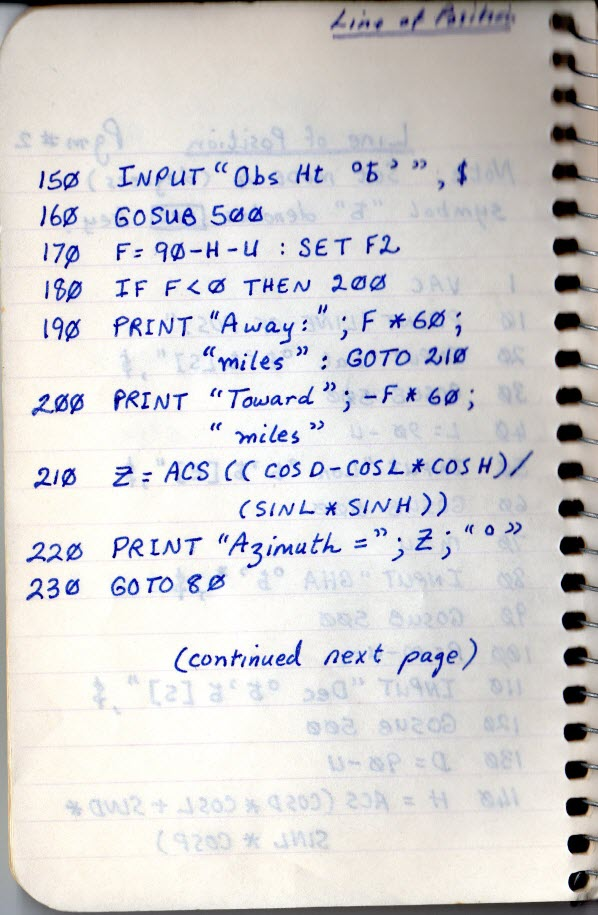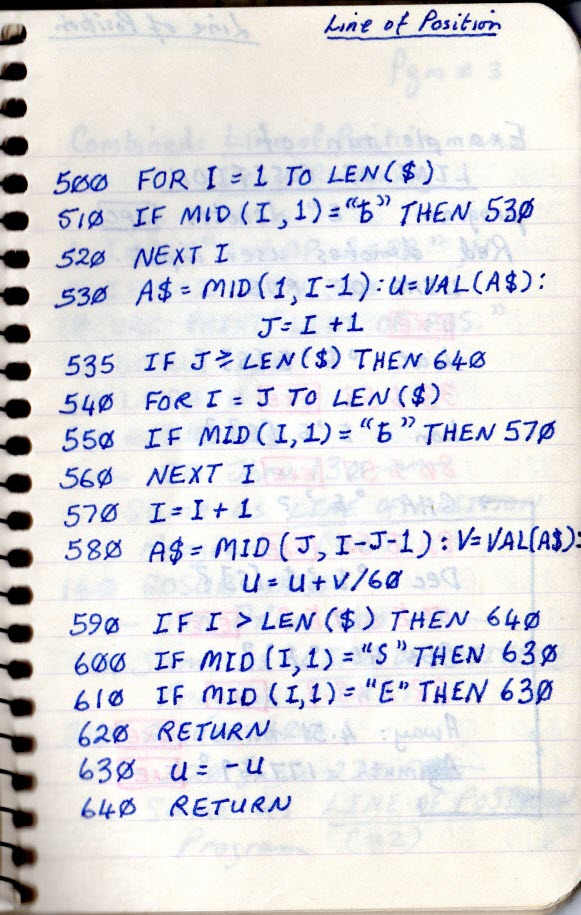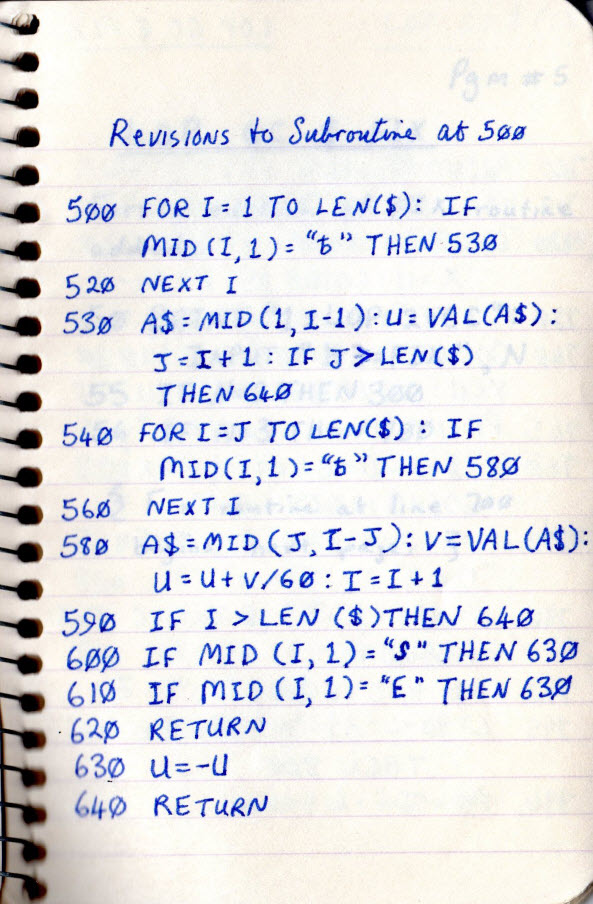Below I have converted the PC-4 Line of Position program into a modern computer BASIC, and included an example run (using data from old notes).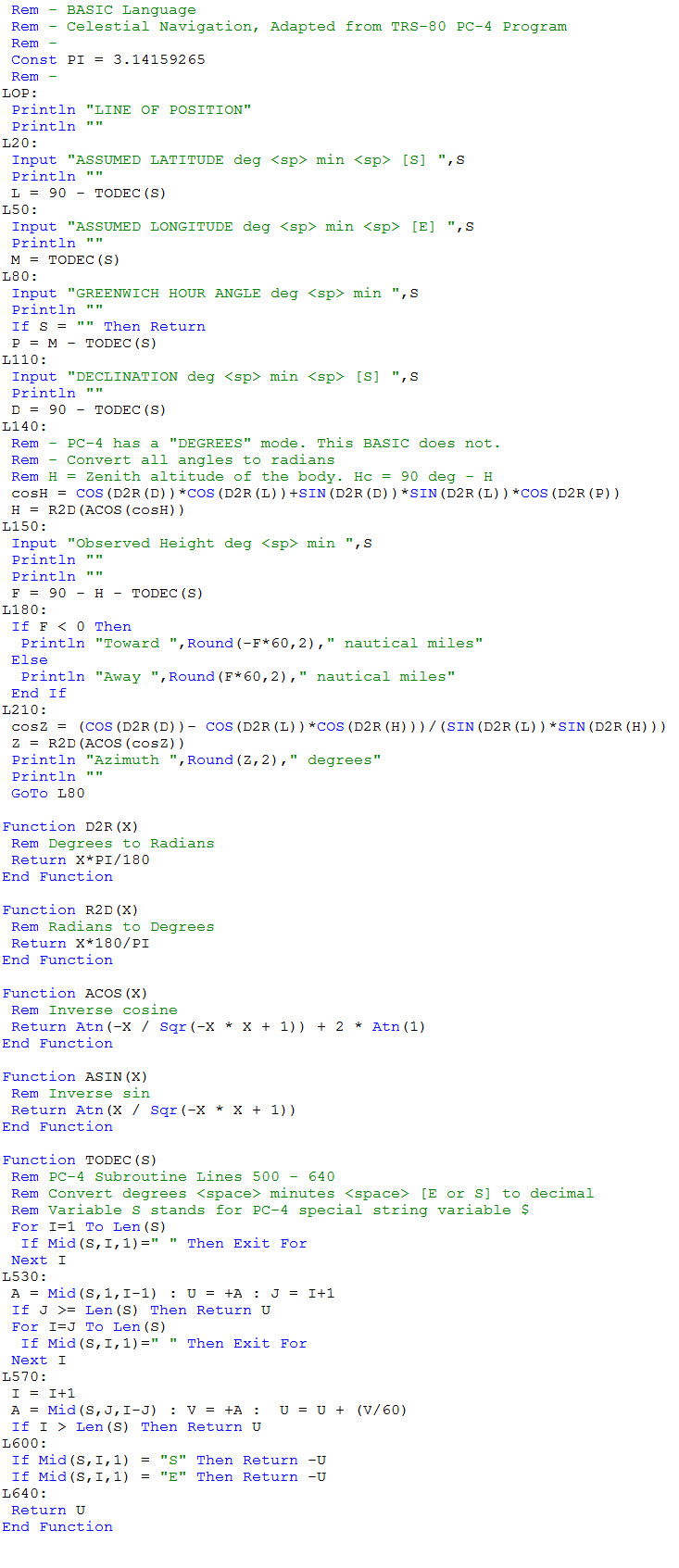This exercise does not improve the PC-4 program.  It is a literal translation, except that input prompts are fully spelled out.

Line labels consisting of the letter “L” and a number correlate to the same-numbered lines of the PC-4 program.

Since the target version of BASIC does not have a “degrees” (calculator) mode, additional functions are included for degrees-to-radians and radians-to-degrees. Similarly the target BASIC had only the arctan function, not arcsin or arccos. Hence, these were also inserted between the main part and the line 500 subroutine part of the program (bottom of listing).

Here is an example run, based on old data.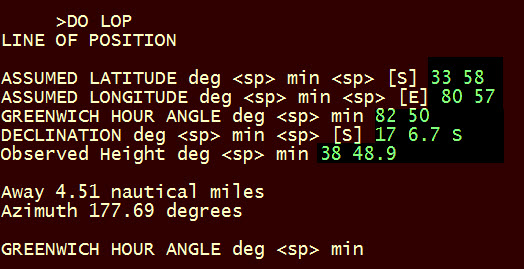I would like to stress how little this program actually does. It computes two things: The first is equation 6 from the parent “Celestial Navigation” page. This is the spherical triangle formula that gives the zenith altitude of the body, from which the computed height (Hc) is immediate.

The second is equation 8, the second spherical triangle formula, which gives the azimuth of the body.

The difference between observed and computed height is the distance toward or away, and the azimuth is the direction of the body.